## Rotational velocity and time related to artificial gravity

Last on our Physics questions for today:

Future space stations and spacecraft may create “artificial gravity” by rotating. Consider a cylindrical space station with a radius of 894 m that rotates about its central axis. The inside surface of the cylinder is the floor of the space station upon which the astronauts live and work. What period of rotation (in s) is necessary for a 70 kg astronaut to have the same weight as he has on Earth?

Now let’s identify what we have:

Astronaut Mass = 70 kg
Gravitational Acceleration (what we want it to be anyhow) = 9.8 m/s^2

The important thing to note is that we need to find the Normal Force being exerted on the astronaut as a result of the angular acceleration.  That force should be the equivalent of the Normal Force on Earth.  That pretty much tells us everything we need to get started.  Now, by this, we can figure the force intended by simple F = ma which gives us 686 N of force.  This is the Normal Force we want to attain.

Now, we need to find out at what velocity the cylinder needs to be spinning in order to obtain 686 N of force.  We can do this by using our angular velocity formula: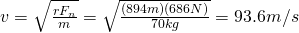Easy as pie right?  Now that we have the velocity, we need to find the total circumference, this will give us the distance that the cylinder has to rotate through in order to complete 1 revolution.Now that we have the total distance and we have velocity, our handy-dandy formula for distance, velocity and time can be used.And there you have it, it would take the space station 60 seconds to make one complete revolution in order to provide the same force on the astronaut as if on Earth.

As always, feel free to correct anything you see wrong with this.

## Tension force related to angular velocity

A question was posed in Physics class:

A toy “rocket-car” is attached to a metal rod that keeps the rocket moving in a horizontal circle on a frictionless table. The radius of the circle is 1.33 m, the mass of the rocket is 0.87 kg, and the rocket engine provides a thrust of 5.7 N. If the rocket starts from rest, how many revolutions will the rocket make before the tension in the rod equals 215 N?

Let’s pick out the important information from the equation:

Attached to a rod moving in a circle.
Radius (of the circle) = 1.33 m
Mass (of the rocket) = 0.87 kg
Thrust (of the engine) = 5.7 N
Final Tension Force = 215 N

Since it is going around in a circle on the table that is frictionless we can ignore gravity for this since it’s only 1 plane of motion.  The important thing to realize is that we need to find the relationship between tension on the arm and thrust of the engine which produces acceleration.  We know that as the object accelerates the tension increases.  We also know that if we can find the total distance traveled and divide it by the distance in one revolution, we’ll get the number of revolutions.

First, we will need to find out exactly how much the object is accelerating.  To get acceleration, we can work backwards since we have the force of thrust as 5.7 N.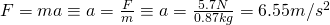Now, we need to figure out what the velocity of the object is when the tension force is 215 N.  To do this, we use the formula for angular force which can be changed to angular velocity: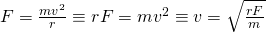This will give us our velocity.  We already know the variables needed, r is 1.33 m, F is the final tension force, 215 N and our mass is 0.87 kg.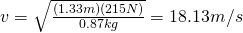Now we have the velocity.  Getting the circumference is the easy part: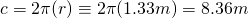Great!  Now we have two more steps.  The first is to use our handy formula that deals specifically with velocity, acceleration and distance values and obtain the total distance:We’re almost done.  Now that we have the total change in distance we can do simple division and we’ll get our answer: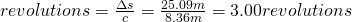And there you have it.  So the total times the object goes around the track before reaching 215 N of force on the arm is 3.

As always, please correct me if you see any problems.  Thanks!

## Minimum velocity related to coefficent of friction

A question posed in Physics class (much help to Judy for the assistance on this one):

One of the rides found at carnivals is the rotating cylinder. The riders step inside the vertical cylinder and stand with their backs against the curved wall. The cylinder spins very rapidly, and at some angular velocity, the floor is pulled away. The thrill-seekers now hang like flies on the wall. If the radius of the cylinder is 2.1 m and the coefficient of static friction between the people and the wall is ms = 0.390, what is the maximum period of rotation (in s) of the cylinder for the floor to be removed safely?

Now, what’s important to note out of all this is that you’re looking to get the angular velocity that will counter the force of gravity enough that static friction is effective in holding the people against the wall.

We know:

Coefficient of static friction = 0.390
Gravitational Acceleration = 9.8 m/s^2

We also know that static friction must be greater than or equal to the force and that must be greater than or equal to 0 in order to remain in place.  Anything less than 0 and the object moves.  So, we need 2 equations for this, one for the force related to gravity and one related to the normal force related to friction: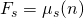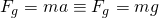Now, the key to this is realizing that the normal force is related, or rather, equal to, the amount of force needed to keep the person in place.  The formula for that is:These can be combined as follows:Now you can plug in numbers for the variables and get an answer for velocity.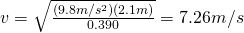Now the second key to this is remembering that the distance around a circle is its circumference, that is the value that you’ll need to put in to get your velocity value spitting out seconds around.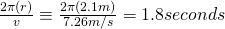And there you have it.  The minimum velocity that the cylinder needs to be spinning is 7.26 m/s which will cause it to rotate once every 1.8 seconds keeping the riders firmly in place.

As always, please correct me if you find any errors.